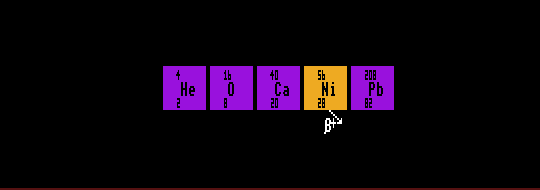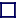The white tramlines outline magic numbers. Isotopes within the horizontal white tram-lines have magic-Z, whilst those within the vertical tramlines have magic-N. Doubly-magic isotopes occur where the tramlines cross (magic-N, magic-Z)

MAGIC NUMBERS

Analogous to electron shells, nuclides with a certain number of neutrons or protons have filled nuclear shells, or closed shells. These numbers are called the nuclear magic numbers, which are 2, 8, 20, 28, 50, 82. However, the shells for protons and shells for neutrons are different from each other, resulting in a different set of magic numbers for protons than for neutrons. Thus for neutrons, the magic numbers are 2, 8, 20, 28, 50, 82, 126 and 184 whilst for protons it is 2, 8, 20, 28, 50, 82, and 114. That is, they are the same numbers until those numbers exceed 100. Nuclides with a magic number of either neutrons or of protons in them are more stable (have a higher binding energy) than surrounding nuclides, but are not necessarily none radioactive. Examples with atomic number, Z, magic are: Z=2 helium; Z=8 oxygen; Z=20 calcium-40; Z=28 nickel-56; Z=50 tin; and Z=82 lead.

Elements with Z magic tend to have a greater number stable isotopes: tin has the most at 10. Nuclides with neutron number, N magic are also more stable and tend to have a greater number of stable isotones. Nuclides with Z or N magic also have much smaller neutron capture cross sections. If both Z and N are magic, then the nuclide is said to be doubly magic, and is even more stable. Such nuclei are spherical with minimum surface area and high stability. Examples are helium-4 (Z=2, N=2); oxygen-16 (Z=8, N=8); calcium-40 (Z=20, N=20); nickel-56 (Z=28, N=28) and lead-208 (Z=82, N=126). Theorists predict that N=184 should be magic and also Z=114 and postulate a stable doubly magic element with Z=114, N=184 and A=298 which has an associated large island of stability. The lifetimes of isotopes in this region are predicted to be up to 30 orders of magnitude longer than they would be in the absence of nuclear shells. There should be a smaller island of stability around the doubly magic nuclide Z=108 and N=162. Also the nuclide Z=102 and N=152 should also be doubly magic. None of these islands has yet been explored experimentally for lack of an experimental method to synthesize such neutron-rich nuclei from lighter nuclei which do not possess enough neutrons between them. A few nuclides to the left of the island of stability at Z=114 N=184 have been synthesized. See Super Heavy Nuclei

Nuclides with an even number of protons are also much more stable than those with an odd number. Similarly, nuclides with an even number of neutrons are more stable that those with an odd number. This is doubly true for nuclides with an even number of both protons and neutrons, being units of helium-4 nuclei (Z=2, N=2). Nuclides favour being made of a whole number of helium-4 nuclei, as in carbon-12, oxygen-16, neon-20, magnesium-24, silicon-28, and sulphur-32, etc, all that is except for beryllium-8, which is so unstable that its halflife is less than 0.2 atto seconds! This helium-4 dependence accounts for the stepwise appearance of the stable elements in the Segre chart.

Note that not all isotopes with doubly-magic numbers of nucleons are stable. It can be seen that isotope Z=50, N=50 is well above the blue stability curve. Tin-100 has a halflife of just 1.1 seconds, and that isotope Z=28, N=50 (nickel-78) is well below the blue stability curve. These simply have either too few neutrons or too many neutrons for stability, respectively.

Shown is helium-4, oxygen-16, calcium-40, nickel-56, and lead-208, all doubly magic.